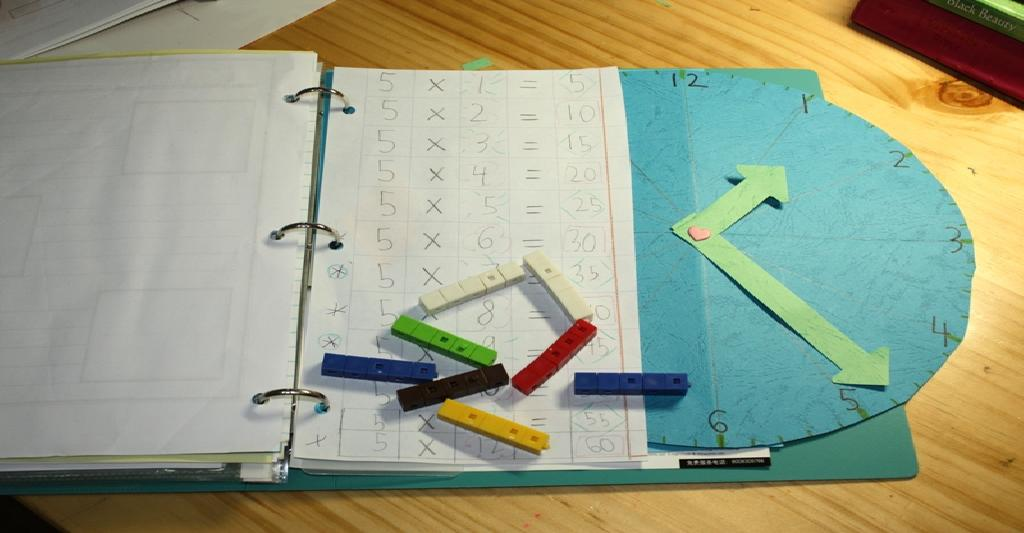# Proof that 1 = 2

Here’s an “interesting” proof I came across. Get ready to rethink everything you knew about Maths:

Given x = 1 and y = 1, then

x = y

Multiplying each side by x,

x2 = xy

Subtracting y2 from each side,

x2y2 = xy – y2

Factoring each side,

(x + y)(x – y) = y(x – y)

Dividing out the common term, (x – y) results in

x + y = y

Substituting the values of x and y,

1 + 1 = 1

or

2 = 1

Q.E.D.

1.Glen Alleman says:
2.Dylan Smith says: